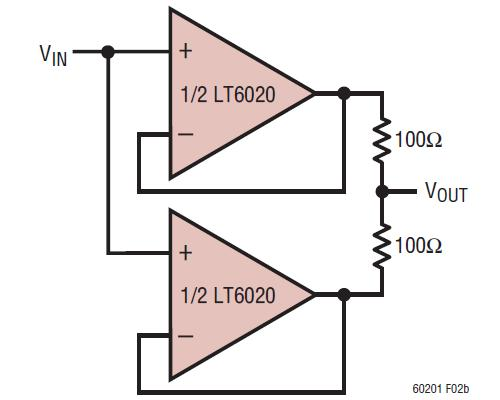# Paralleling Amplifiers Improves Signal-to-Noise Performance

Dealing with low amplitude signals can be challenging. In order to differentiate between the low level signal and the noise contributed by surrounding circuitry, we typically use gain to amplify the signal above the noise floor. However, a standard amplifier configuration multiplies the input signal, the input noise, and the noise contribution of the amplifier by the gain of the amplifier. So while the amplitude of the input signal gets larger, so does the input noise and the noise added by the amplifier, resulting in no improvement to the signal-to-noise ratio (SNR).

There is a way to use an amplifier to not only increase the signal amplitude, but to improve the SNR as well. The key to this approach is to use the amplifier in a summing configuration, with an understanding of the difference between correlated and uncorrelated signals. In the summing configuration, because we are summing two uncorrelated noise sources (the amplifier noise), the amplifier noise increases as √2, while the correlated input signal amplitude increases by 2. This principle can be extended to N amplifiers for signifcant SNR improvement.

Mathematically, with a single amplifier having an input signal SIN and gain G, the total combined input signal is given by  the signal (SIN) plus any externally generated noise at the input (NIN); this is written as SIN + NIN. The output includes the output signal (SOUT) plus the total output noise (NOUT); these can be written as SOUT = SIN*G and NOUT = NIN*G + NAMPL1, respectively, where NAMPL1 is the output-referred noise due to the amplifier itself.

The SNR is calculated by dividing the output RMS signal power by the output RMS noise power. This comes out to be:

SNR (one amplifier) =  (SOUT)2 / (NOUT)2 = (SIN*G)2 / ((NIN*G)2 + NAMPL12)

Assuming the external noise input power is minimal (for simplicity) reduces the equation to (SIN*G)2 / NAMPL12.

Now, adding a second amplifier in parallel increases the RMS signal power by 2x, but only increases the RMS amplifier noise by √2 because the amplifiers add uncorrelated noise. So instead of the noise doubling (NAMPL1 plus NAMPL2), we obtain a noise of √2*NAMPL.The SNR calculation for two amplifiers in parallel is:

SNR (two amplifiers) = (2SIN*G)2 / ((2NIN*G)2 + (√2((NAMPL1)2 + (NAMPL2)2))) = (2SIN*G)2 / ((2NIN*G)2 + (√2NAMPL)2)  = (SIN*G)2 / ((NIN*G)2 + 0.5*(NAMPL2)).

Again, assuming the external input noise power is minimal reduces this to (SIN*G)2 / (0.5*(NAMPL2))

The benefits of adding amplifiers in parallel are improved SNR and lower voltage noise density. For N amplifiers in parallel, the amplifier noise power is reduced by N and the input referred voltage noise density is reduced by √N. Put another way, each time the number of amplifiers is doubled, the amplifier noise power decreases by 2 and the amplifier's input referred voltage noise density decreases by √2. Note that paralleling amplifiers can only reduce the uncorrelated noise added by the amplifiers; it cannot reduce the noise due to other external noise sources (ex. resistors, sensors, other signal conditioning components, etc. In the two parallel amplifier circuit above, the LT6020's input voltage noise density is reduced from 45nV/√Hz to 32nV/√Hz.

The circuit below reveals how the input noise density is reduced for N amplifiers with the LT1028 ultralow 0.85nV/√Hz noise density, precision amplifier.The paralleling amplifiers technique can be extended to composite amplifier designs, where the two amplifiers each provide a unique benefit to the circuit. An example of this is found in Design Note 241; the circuit is shown below. Here, two LT1806's are used to provide a low distortion, high output drive circuit solution; one amplifier supplies the 50 ohm cable drive capability while the other provides the precision.Another advantage of using parallel amplifiers is the output offset error is reduced. Since offset error is not correlated, as one adds more amplifiers, the distribution of offset errors will move toward a center value, such that the net value when all devices are considered will approach the typical mean value.When calculating noise, keep in mind current noise, resistor noise (feedback resistors, source impedance, etc.) and external noise must all be taken into consideration. One consequence of using amplifiers in parallel is that current noise increases. Other trade-offs include cost and component count. These however often can be justified by the improved performance obtained using the paralleled amplifiers technique.